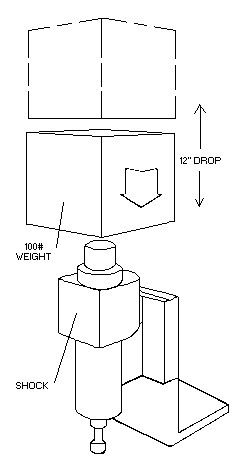LINEAR SHOCK ABSORBERE = WH
E = 100 x 12″
E = 1200 INCH LBS.

SYMBOLS

E = Energy                                       Inch Pounds
W = Weight                                     Pounds
V = Contact Velocity                       Ft/Sec.
Note: Contact Engineering Department is contact velocity exceeds 30 ft./sec.
H = Total Vertical Distance           Inches
T = Deceleration Time                   Sec
D = Shock Absorber Stroke           Inches
G = Deceleration Force                  Pounds
F = Driving Force                           Pounds
T = Propelling Torque                    Inch Pounds